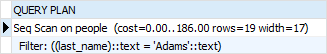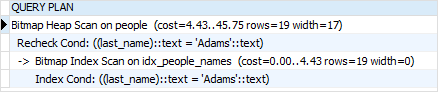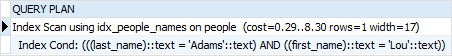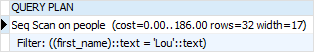# PostgreSQL Tutorial: Multicolumn Indexes

August 2, 2023

Summary: in this tutorial, you will learn how to create multicolumn indexes which are indexes defined on more than one column of a table.

## Introduction to PostgreSQL multicolumn indexes

You can create an index on more than one column of a table. This index is called a multicolumn index, a composite index, a combined index, or a concatenated index.

A multicolumn index can have a maximum of 32 columns of a table. The limit can be changed by modifying the `pg_config_manual.h` when building PostgreSQL.

In addition, only B-tree, GIST, GIN, and BRIN index types support multicolumn indexes.

The following syntax shows how to create a multicolumn index:

``````CREATE INDEX index_name
ON table_name(a,b,c,...);
``````

When defining a multicolumn index, you should place the columns which are often used in the `WHERE` clause at the beginning of the column list and the columns that are less frequently used in the condition after.

In the above syntax, the PostgreSQL optimizer will consider using the index in the following cases:

``````WHERE a = v1 and b = v2 and c = v3;
``````

Or

``````WHERE a = v1 and b = v2;
``````

Or

``````WHERE a = v1;
``````

However, it will not consider using the index in the following cases:

``````WHERE  c = v3;
``````

or

``````WHERE b = v2 and c = v3;
``````

## PostgreSQL Multicolumn Index example

To demonstrate multicolumn indexes, we will create a new table named `people` with three columns: id, first name, and last name:

``````CREATE TABLE people(
id INT GENERATED BY DEFAULT AS IDENTITY,
first_name VARCHAR(50) NOT NULL,
last_name VARCHAR(50) NOT NULL
);
``````

You can use the following script to load 10,000 rows into the `people` table:

The following statement finds people whose last name is `Adams`:

``````SELECT
*
FROM
people
WHERE
``````

Here is the output:As shown clearly in the output, PostgreSQL performed the sequential scan on the `people` table to find the corresponding rows because there was no index defined for the `last_name` column.

Let’s define a B-tree index on both `last_name` and `first_name` columns. Assuming that searching for people by their last name is more often than by their first name, we define the index with the following column order:

``````CREATE INDEX idx_people_names
ON people (last_name, first_name);
``````

Now, if you search for people whose last name is `Adams`, the PostgreSQL optimizer will use the index as shown in the output of the following statement:

``````EXPLAIN SELECT
*
FROM
people
WHERE
``````

The output is:The following statement finds the person whose last name is `Adams` and the first name is `Lou`.

``````SELECT
*
FROM
people
WHERE
AND first_name = 'Lou';
``````

The following shows the output:The PostgreSQL Optimizer used the index for this statement because both columns in the `WHERE` clause are all in the index:

``````EXPLAIN SELECT
*
FROM
people
WHERE
AND first_name = 'Lou';
``````

However, if you search for people whose first name is `Lou`, PostgreSQL will perform a sequential scan of the table instead of using the index as shown in the output of the following statement:

``````EXPLAIN SELECT
*
FROM
people
WHERE
first_name = 'Lou';
``````Even though the `first_name` column is a part of the index, and PostgreSQL could not leverage it.

A key point to take away is that when you define a multicolumn index, you should always consider the business context to find which columns are often used for lookup and place these columns at the beginning of the column list while defining the index.

In this tutorial, you have learned how about the PostgreSQL multicolumn index and the importance of the column order in the multicolumn indexes.